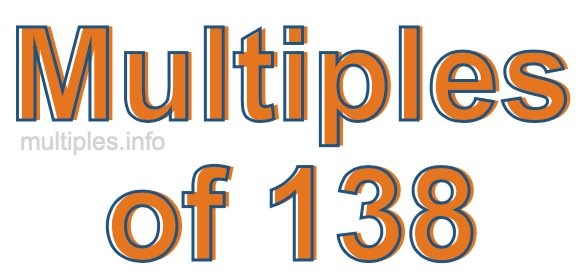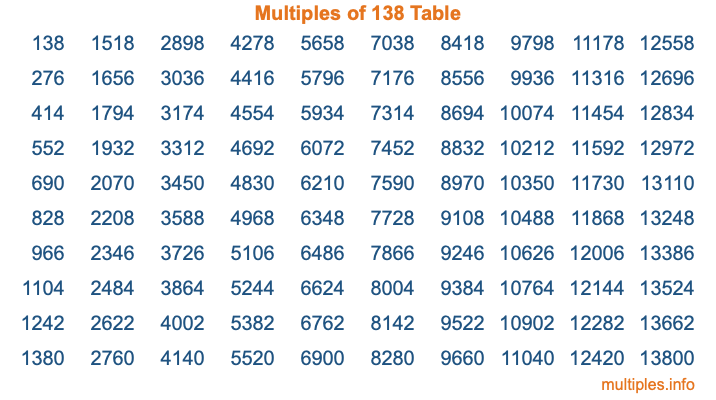Multiples of 138Welcome to the Multiples of 138 page. Here we will first teach you everything you will ever need to know about the multiples of 138, and then give you a study guide summary of everything we taught you to make sure you remember it all. Use this page to look up facts and learn information about the multiples of 138. This page will make you a multiples of one hundred thirty-eight expert!

Definition of Multiples of 138
Multiples of 138 are all the numbers that when divided by 138 equal an integer. Each of the multiples of 138 are called a multiple. A multiple of 138 is created by multiplying 138 by an integer.

Therefore, to create a list of multiples of 138, you start with 1 multiplied by 138, then 2 multiplied by 138, then 3 multiplied by 138, and so on for as long as you want. Thus, the list of the first five multiples of 138 is 138, 276, 414, 552, and 690. To see a larger list of multiples of 138, see the printable image of Multiples of 138 further down on this page. We also have a category where you can choose any nth multiple of 138.

Multiples of 138 Checker
The Multiples of 138 Checker below checks to see if any number of your choice is a multiple of 138. In other words, it checks to see if there is any number (integer) that when multiplied by 138 will equal your number. To do that, we divide your number by 138. If the the quotient is an integer, then your number is a multiple of 138.

Is  a multiple of 138?

Least Common Multiple of 138 and ...
A Least Common Multiple (LCM) is the lowest multiple that two or more numbers have in common. This is also called the smallest common multiple or lowest common multiple and is useful to know when you are adding our subtracting fractions. Enter one or more numbers below (138 is already entered) to find the LCM.

Check out our LCM Calculator if you need more details about the Least Common Multiple or if you need the LCM for different numbers for adding and subtraction fractions.

nth Multiple of 138
As we stated above, 138 is the first multiple of 138, 276 is the second multiple of 138, 414 is the third multiple of 138, and so on. Enter a number below to find the nth multiple of 138.

th multiple of 138

Multiples of 138 vs Factors of 138
138 is a multiple of 138 and a factor of 138, but that is where the similarities end. All postive multiples of 138 are 138 or greater than 138. All positive factors of 138 are 138 or less than 138.

Below is the beginning list of multiples of 138 and the factors of 138 so you can compare:

Multiples of 138: 138, 276, 414, 552, 690, etc.

Factors of 138: 1, 2, 3, 6, 23, 46, 69, 138

As you can see, the multiples of 138 are all the numbers that you can divide by 138 to get a whole number. The factors of 138, on the other hand, are all the whole numbers that you can multiply by another whole number to get 138.

It's also interesting to note that if a number (x) is a factor of 138, then 138 will also be a multiple of that number (x).

Multiples of 138 vs Divisors of 138
The divisors of 138 are all the integers that 138 can be divided by evenly. Below is a list of the divisors of 138.

Divisors of 138: 1, 2, 3, 6, 23, 46, 69, 138

The interesting thing to note here is that if you take any multiple of 138 and divide it by a divisor of 138, you will see that the quotient is an integer.

Multiples of 138 Table
Below is an image of the first 100 multiples of 138 in a table. The table is in chronological order, column by column. The first column has the first ten multiples of 138, the second column has the next ten multiples of 138, and so on.The Multiples of 138 Table is also referred to as the 138 Times Table or Times Table of 138. You are welcome to print out our table for your studies.

Negative Multiples of 138
Although not often discussed or needed in math, it is worth mentioning that you can make a list of negative multiples of 138 by multiplying 138 by -1, then by -2, then by -3, and so on, to get the following list of negative multiples of 138:

-138, -276, -414, -552, -690, etc.

Multiples of 138 Summary
Below is a summary of important Multiples of 138 facts that we have discussed on this page. To retain the knowledge on this page, we recommend that you read through the summary and explain to yourself or a study partner why they hold true.

There are an infinite number of multiples of 138.

A multiple of 138 divided by 138 will equal a whole number.

138 divided by a factor of 138 equals a divisor of 138.

The nth multiple of 138 is n times 138.

The largest factor of 138 is equal to the first positive multiple of 138.

138 is a multiple of every factor of 138.

138 is a multiple of 138.

A multiple of 138 divided by a divisor of 138 equals an integer.

138 divided by a divisor of 138 equals a factor of 138.

Any integer times 138 will equal a multiple of 138.

Multiples of a Number
Here you can get the multiples of another number, all with the same attention to detail as we did for multiples of 138 on this page.

Multiples of
Multiples of 139
Did you find our page about multiples of one hundred thirty-eight educational? Do you want more knowledge? Check out the multiples of the next number on our list!